Ch 2. Fluid Statics Multimedia Engineering Fluids PressureVariation PressureMeasurement HydrostaticForce(Plane) HydrostaticForce(Curved) Buoyancy
 Chapter 1. Basics 2. Fluid Statics 3. Kinematics 4. Laws (Integral) 5. Laws (Diff.) 6. Modeling/Similitude 7. Inviscid 8. Viscous 9. External Flow 10. Open-Channel Appendix Basic Math Units Basic Equations Water/Air Tables Sections Search eBooks Dynamics Fluids Math Mechanics Statics Thermodynamics Author(s): Chean Chin Ngo Kurt Gramoll ©Kurt GramollFLUID MECHANICS - THEORY

In this section, the discussion focuses on determining the buoyant force exerted by the fluid on a submerged or floating body. It is also desired to determine what factors affect the stability of the body.

Buoyancy - Archimedes' Principle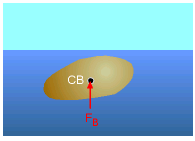Totally Submerged Body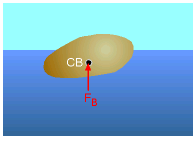Partially Submerged / Floating Body

When a body is totally or partially submerged in a fluid, a resultant force acts on the body pushing the body upward. This force is called the buoyant force. Its magnitude is given by the weight of the fluid displaced by the body. That is,

 FB = ρgV

where ρ is the density of the fluid and V is the displaced volume.

The direction of this buoyant force is upward, and it passes through the center of gravity (CG) of the displaced volume (not the CG of the object itself). This point is called the center of buoyancy (CB). This is known as Archimedes' principle.

For a partially submerged or floating body, the weight displaced by the fluid above the liquid surface (usually air) is relatively small compared to the weight displaced by the liquid, hence it can be neglected.

Stability of Submerged or Floating Bodies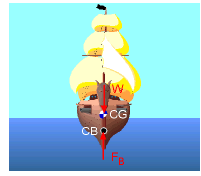A Ship in Equilibrium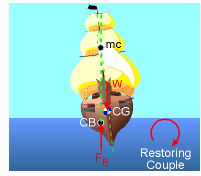Stable Equilibrium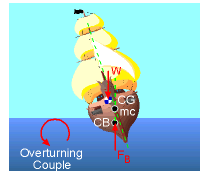Unstable Equilibrium

A completely submerged body is said to be in stable equilibrium as long as its CG (not the CG of the displaced volume) is directly below the CB. In the event when CG coincidents with CB, the body is said to be in neutral equilibrium. If the CG is above the CB then the body may be unstable, and the problem requires further analysis.

Stability issues are great concerns in the design of ships (a partially submerged body). Consider a ship in an equilibrium condition, as shown in the figure, such that CG is directly above CB. If the ship is tipped, the location of CB is shifted due to the change in the displaced volume; if the ship is located such that CB is at the left of CG, the buoyant force and the weight will form a couple. The tendency of this couple is to restore the ship to its original equilibrium position, hence the ship is said to be in stable equilibrium. If the ship is tipped such that CB is at the right of CG, the produced couple tends to capsize the ship and the ship is now in an unstable equilibrium position.

Another way to determine the stability of a floating body is through the concept of the metacenter. The metacenter (mc) is the point of intersection of the vertical centerline axis of the body and the line of action of the buoyant force. It can be observed from the figures that when the mc is above CG, a restoring couple is produced and the floating body is in stable equilibrium; whereas when the mc is below CG, an overturning couple is produced and the body is in unstable equilibrium.

Practice Homework and Test problems now available in the 'Eng Fluids' mobile app
Includes over 250 problems with complete detailed solutions.
Available now at the Google Play Store and Apple App Store.